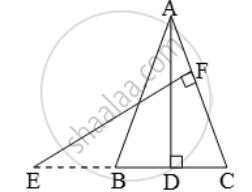# In the figure, E is a point on side CB produced of an isosceles ∆ABC with AB = AC. If AD ⊥ BC and EF ⊥ AC, prove that ∆ABD ~ ∆ECF - Mathematics

Sum

In the figure, E is a point on side CB produced of an isosceles ∆ABC with AB = AC. If AD ⊥ BC and EF ⊥ AC, prove that ∆ABD ~ ∆ECF#### Solution

Given: A ∆ABC in which AB = AC and AD ⊥ BC. Side CB is produced to E and EF ⊥ AC.

To prove: ∆ABD ~ ∆ECF.

Proof: we known that the angles opposite to equal sides of a triangle are equal.

∠B = ∠C [∵ AB = AC]

Now, in ∆ABD and ∆ECF, we have

∴ ∠B = ∠C            [proved above]

∴ ∆ABD ~ ∆ECF    [By AA-similarity]

Concept: Similarity
Is there an error in this question or solution?